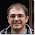## Monday, June 18, 2012

This is a simple formula to calculate the mac-address for checkpoint loadsharing on multicast mode. This step is important as without configuring a static arp, most of the Cisco routers/switches that is interconnecting with the firewall cluster will not function.

Taking the following formula into consideration:
Divide the Ip address into 4 octets as follow:

x.y.z.w

If  y <=127
then the mac address will be 01:00:5e:y:z:w
Simply convert the y:z:w from decimal to Hex

example:
10.1.1.1
then the mac adress will be 01:00:5e:01:01:01

if y > 127
then the mac address will be  01:00:5e:(y-128):z:w
Use the value for y minus 128 then convert them from decimal to Hex.

Example:
10.157.1.1
then mac address will be 01:00:5e:(157-128):z:y = 01:00:5e:1d:01:01

#### 1 comment:

1.Thanks for explanation!

I think your example 10.1.1.1 should be 0100.5e01.0101, not 0100.5e0a.0101